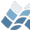NV5 GEOSPATIAL SOFTWARECNB_IMF

## Purpose

This function both evaluates the IMF at given mass, and returns
masses drawn at random from the IMF. The functional form of the IMF
is given by dN/dM ~ M^-alpha, where
alpha = 0 for 0 < M / Msolar < .07 (Allen et al 2005)
= 1.05 for .07 < M / Msolar < .5 (Kroupa et al 2002 ASPC..285...86K)
= 2.35 for .5 < M / Msolar (Ibid)

Astrophysics

## Calling Sequence

result = cnb_imf([mass, /noturnover, random = random, muench = muench)

## Inputs

mass: A set of masses at which to evaluate the IMF. Given in solar
masses. If provided, the program returns imf(masses) as a
scalar or vector. This function is not normalized

## Keyword Parameters

NOTURNOVER: If set, treat the full IMF as having alpha = 2.35. This
cannot be used in conjunction with the RANDOM keyword
RANDOM: Set this to a number (nstars) to draw nstars from the IMF
mass distribution. If this keyword is set, then the function
returns the masses of the nstars drawn from the distribution.
MUENCH: Use the first three terms of the power law imf given in
equation 1 of Muench et al. 2002 (ApJ 573)
MALPHA0: Manually specify the high mass value for alpha.

## Outputs

If mass is set, it returns a vector of imf(mass). If random is set,
it returns a vector of masses drawn from the IMF distribution.

## Modification History

April 2009: Written by Chris Beaumont
May 2009: Added RANDOM keyword. cnb.
June 2009: Added MUENCH keyword. cnb.

© 2023 NV5 Geospatial |  Legal